Open In App

# How to find the Perimeter of a Trapezoid?

A trapezoid is a flat, closed shape with four straight faces and a pair of parallel faces. Parallel sides of a trapezoid are called bases, and nonparallel sides are called legs. A trapezoid can also have parallel sides. Parallel planes can be horizontal, vertical, or inclined.

### Trapezoid

A trapezoid is a polygon with only one pair of parallel sides. This parallel side is also called the parallel base of the trapezoid. The other two sides of the trapezoid are not parallel and are called the legs of the trapezoid. One school of mathematics asserts that a trapezoid can have a pair of parallel sides, while another school of mathematics asserts that a trapezoid can have more than one pair of parallel sides. Considering the second definition, a parallelogram is also trapezoidal accordingly. However, the first definition does not consider a parallelogram to be a trapezoid.

Types Of Trapezoid

Trapezoids can be divided into three categories,

• Right Trapezoid: A right-angled trapezoid is a trapezoid in which a pair of right angles are adjacent to each other.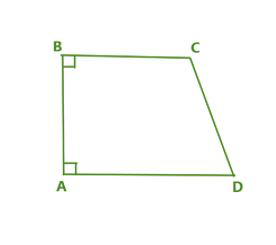• Isosceles Trapezoid: If the nonparallel sides or legs of a trapezoid are the same length, they are said to be an isosceles trapezoid.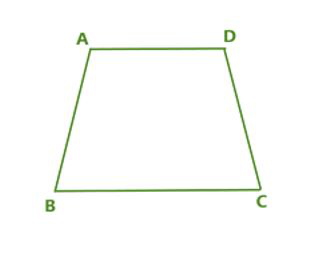• Scalene Trapezoid: If the sides and angles of a trapezoid are not equal, we call it a scalene trapezoid.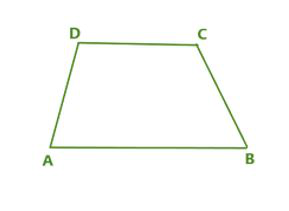### Perimeter Of Trapezoid

The perimeter of a trapezoid is equal to the sum of the lengths of the sides of the trapezoid. The formula for the perimeter of a trapezoid is as simple as the sum of the lengths of its four sides. In the trapezoid ABCD below, consider the case where sides AB and CD (base) are parallel to each other, but sides AD and BC (legs) are not. The perimeter of a trapezoid ABCD can be calculated using the formula perimeter (P) = AB + BC + CD + DA. It can also be expressed as the sum of the lengths of parallel sides and the sum of the lengths of non-parallel sides. where AB and CD are parallel sides and AD and BC are nonparallel sides.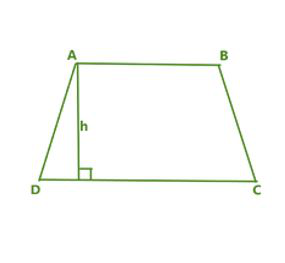### How to find the perimeter of a trapezoid?

The perimeter of a trapezoid can be calculated by following these steps:

Step 1: Record the dimensions of all sides of the trapezoid.

Step 2: Add the lengths of all sides.

Step 3: Once the perimeter value is obtained, record the units with this value.

Example: What is the perimeter of the trapezoid when the nonparallel sides are 10 units long and the parallel sides are 5units long?

Solution:

Suppose the sum of the lengths of the nonparallel sides = 10 units, then the sum of the parallel sides = 5 units. The perimeter of a trapezoid = Sum of the lengths of parallel sides + Sum of lengths of non-parallel sides ⇒ P = 10 units + 5 units.

So, perimeter (P) = 15 units.

### How to find the perimeter of a Trapezoid with a missing side?

The perimeter of a trapezoid can be calculated even if there are not enough sides. In such cases, you can use the given sides of the trapezoid, apply the Pythagoras theorem and other properties to find the missing sides, and then calculate the perimeter.

Example: Find the perimeter of the trapezoid PQRS with dimensions  PQ = 110m, SV = 40m, VU = 110m, UR = 70m, QU = 80m.

Solution

Use the given dimensions to find the missing PS and QR faces.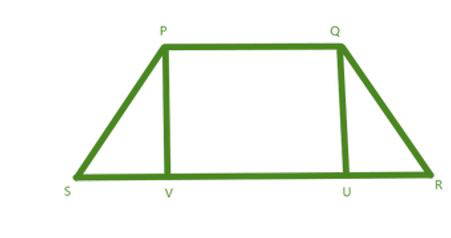Step 1: If we take the △QUR, we get QU = 80m and UR = 70m. We can see that △QUR is a right-angled triangle. So we can use the Pythagoras theorem to calculate the QR value.

Step 2: According to the Pythagoras theorem, QR2 = QU2 + UR2. This means QR2 = 802 + 702. So QR2 =  6400 +4900 ⇒ QR = √11300 = 106.301m.

Step 3: Since QU = 80m,  PQ is parallel to SR, so PV is also 80m, so we can now calculate the missing side PS of the trapezoid.

Step 4: Taking the right triangle △PSV,  PV = 80m, SV = 40m, so applying the Pythagoras theorem we get PS2 = PV2 + SV2. This means PS2 = 802 + 402. So, PS2 = 6400 + 1600 ⇒ PS = √8000 = 89.44m.

Step 5: Now that we know all the faces of the trapezoid, we can add up all four faces to find the perimeter. This means that the perimeter of the trapezoid is PQRS = PQ + QR + RS + SP ⇒ 110 + 106.301 + 220 + 89.44 = 525.741 m.

### Sample Questions

Question 1: Give two basic trapezoidal formulas.

Perimeter  of Trapezoid = sum of all sides of trapezoid = a + b + c +d

Area of Trapezoid = A = [(a + b)/2] × h.

Where a , b , c , d are length of four sides of trapezoid.

Question 2: Is a trapezoid / trapezoid considered a quadrilateral?

A quadrilateral is a closed shape having four sides. As a result,  trapezoids are sometimes called quadrilaterals. Some people describe a trapezoid as a quadrilateral with only one set of parallel sides and remove the parallelogram from the definition. Others define a trapezoid as a quadrilateral with at least one pair of parallel sides, making a parallelogram a particular type of trapezoid. Trapezoids come in different sizes of angles.

Question 3: What is the difference between a trapezoid and a trapezium?

A trapezium is a quadrilateral with a pair of parallel sides facing each other, trapezoid is also a quadrilateral polygon with one of its parallel sides opposite to each other.

So, the two terms trapezoid and trapezium are the same but have different spellings in different countries. In American English, it is trapezium but in British English it’s a trapezium.

Question 4: Give three properties of a trapezoid.

• If the trapezoid is isosceles, then its base and diagonal are equal.
• The intersection of the diagonals lies on the same line as the midpoint of the two opposite sides.
• The opposite sides of an isosceles trapezoid are the same.

Question 5: Are the diagonals of a trapezoid equal? Explain.

There are three types of trapezoids: isosceles, multipurpose, and straight. For an isosceles trapezoid, the diagonals are the same because the non-parallel sides or legs of a trapezoid are of the same length. For scalene and straight trapezoid, the diagonals are not equal.

Question 6: If the perimeter of the trapezoid is 60 units and the three sides are 10 units, 25 units, and 12 units, respectively, use the trapezoidal formula to find the size of the fourth side.

Solution:

• Given perimeter of trapezoid = 60 units
• Given length of side 1 of trapezoid =10 units
• Given length of side 2 of trapezoid = 25 units
• Given length of side 3 of trapezoid = 12 units

Let d units be the length of fourth side of trapezoid,

Using trapezoid formula , Perimeter of trapezoid = Sum of all sides of trapezoid

60 = 10 + 25 + 12 +d

60 = 47 + d

d = 13 units.